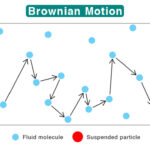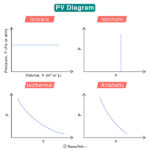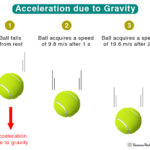Home / Physics / Thermal Energy

# Thermal Energy

Matter consists of atoms, molecules, and electrons that constantly vibrate and move. Thermal energy is the energy due to the motion of atoms and molecules in a substance. It accounts for translational, vibrational, and rotational motion. Since it involves the random movement of molecules, thermal energy is a type of kinetic energy. It can explain how matter transforms from one state to another.

English physicist and mathematician James Prescott Joule discovered thermal energy in 1847.

## How is Thermal Energy Produced

Temperature measures the average kinetic energy of the molecules. When the temperature rises, the molecules become agitated and collide faster, resulting in thermal energy. The hotter the substance, the faster the molecules travel, and higher is the thermal energy. On the other hand, the molecules slow down and lower the thermal energy when the temperature is reduced.

## What is the Difference Between Thermal Energy and Heat

Another word for thermal energy is heat energy, not to be confused with heat. There is a difference between thermal energy and heat. While thermal energy refers to the motion of particles in a substance, heat refers to the flow of thermal energy. It happens when there is a temperature gradient in the substance. Heat flows from a higher temperature to a lower temperature. Unlike thermal energy, heat is not a property of the substance.

## Thermal Energy Transfer

Thermal energy transfers in three different ways.

1. Conduction: A process through which thermal energy is transferred between two molecules in contact. The transfer occurs when molecules strike one another, resulting in collisions. Conduction takes place in solid, liquid, and gas. For example, when we heat one end of a metal rod, thermal energy quickly transfers to the other end.

2. Convection: It occurs when thermal energy is transferred through a medium like liquid or gas. Molecules carry the energy from a hot region to a cold region. For example, when we boil water in a pan, the molecules at the bottom get heated first and carry the energy to the top.

3. Radiation: It is the process by which energy is transferred without contact between the molecules. No medium is necessary for the energy to travel as electromagnetic waves carry it. An example of radiation is sunlight which is essential for all living beings on Earth. The energy received from the sun is known as solar thermal energy. It is renewable.

## Examples of Thermal Energy

Here are some examples where thermal energy is emitted or transferred in everyday life.

• Stove, microwave oven, toaster, and heater are sources of thermal energy
• A cup of hot tea or a slice of hot pizza radiates thermal energy
• A glass of water transfers thermal energy to an ice floating on it
• A bathtub filled with hot water, a hot water pool, and a spa conducts thermal energy
• Convection currents carry thermal energy in the atmosphere

Conversion of Chemical Energy into Thermal Energy

• Burning of coal, natural gas, and gasoline
• An explosion
• Combustion and exothermic reactions
• Hand warmers
• Butane torch

Conversion of Electrical Energy into Thermal Energy

• Heater
• Microwave oven
• Heat pump
• Toaster
• Coffee making machine

An example of a device that converts thermal energy into electrical energy is a thermoelectric generator.

## How to Calculate Thermal Energy

Consider a man pushing a box, which is in contact with the floor. As a result, there will be friction between the box and the floor. The man applies force and displaces the box over a specific distance. The floor exerts a friction force on the box such that it is equal to the applied force. The box moves with a constant velocity as there is no net force. The work done by the man in displacing the box is given by

W = F · d

Or, W = f · d

Where

W : Work done

F: Applied force

d : Displacement of the box

f : Friction force

From the law of conservation of energy, work done by the man is converted into thermal energy. Heat is generated in the region between the box and the floor, which increases the temperature. The equation can be written as follows.

Change in thermal energy = Work done

Or, ΔET = f · d

Where

ΔET : Change in thermal energy

SI Unit: Joule or J (1 J = 1 kg m2/s2)

The friction force is given by

f = μKFN

Where

μK : Coefficient of kinetic friction

FN : Normal force

The normal force can be written in terms of the box’s mass.

FN = mg

Where

m : Mass of the box

g : Acceleration due to gravity

Therefore

ΔET = μKmg

The example above shows how mechanical energy (man pushing a box) can be converted into thermal energy. It is also possible to convert thermal energy into mechanical energy by using a heat engine.

## Applications of Thermal Energy

• It is the source of power in thermal power plants.
• It can be stored and used as a power supply during peak hours.
• It can be used to heat food, boil water, and heat homes
• Thermal energy due to Earth is known as geothermal energy. It is a renewable source of energy that can supply electricity to homes.
• Car engines convert thermal energy into mechanical energy

## FAQs

Q.1. How does mass affect thermal energy transfer?

Ans. If the temperature remains constant, increasing an object’s mass increases its thermal energy.

Q.2. How does the type of material affect thermal energy transfer?

Ans. Suppose two substances have the same mass and temperature. In that case, the one with higher specific heat will have more thermal energy.

Q.3. Can graphene conduct thermal energy?

Ans. Graphene is an excellent conductor of thermal energy

Article was last reviewed on Wednesday, March 23, 2022

### Related articlesBrownian MotionPV DiagramVelocity vs AccelerationAcceleration due to Gravity